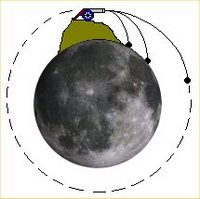## Saturday, October 29, 2005

### Newton's Cannon on the MoonOne of the things I'm working on now is a teacher's guide for Orbiter. It's not too far along, in part because I'm still hoping to find a real science teacher to work with me on it -- I think Orbiter can be a valuable tool in classrooms from grade 8 and up, and I think I have some good ideas, but never having been a teacher, I want to make sure the guide is grounded in school reality.

One thing the guide will have is some suggested lessons and demos, and one could explore the basic question "how do orbits work?" A classic explanation was first used by Sir Isaac Newton in his Principia, "Newton's mountain" (here is a cool interactive Java applet version). The thought experiment starts with a very tall mountain, tall enough to be above most of the Earth's atmosphere. A very powerful cannon fires cannonballs horizontally, adding powder each time to make the ball go faster. Slower balls hit the Earth, but at a certain speed, the curving slope of the Earth's surface "out-races" the cannonball's curved path, and the ball is in orbit.

In Orbiter, this experiment can be done on the Moon (Newton also worked out the orbital motion of the Moon in his Principia - it was the only Earth satellite around at the time). I use the Delta Glider (DG), which features separate main and hover engines. I first decide on an orbital height, say 20 km (low but OK, the Moon has no air to worry about) . I then calculate the circular orbital speed for this height (which is equivalent to R=1758 km from the Moon's center). The formula is Sqrt(G*M/R), where G is the universal gravitational constant (not the Moon's g), M is the mass of the Moon, and R is the distance from the Moon's center (Sqrt means square root). This works out to 1671 meters/second.

To get around the problem of gradual acceleration (Newton's imaginary cannon effectively has infinite acceleration, but Orbiter's spacecraft do not), I decided to have the the spacecraft accelerate up to a reference point (Orbiter's standard Moon base, Brighton Beach), using automatic hover to hold the 20 km altitude, and cutting the main and hover engines just over the base. I give the spacecraft unlimited fuel so its mass will not change as it accelerates, and I test and find that its main engine gives acceleration a=12.3 m/s^2. So to reach 1671 m/s, I need to accelerate for t = v/a = 1671/12.3 or 135.85 seconds.

But how far away should I start? There's another high school physics formula for this, for acceleration a, time t, distance d, d = (1/2)*a*t^2 (time squared). This works out to 113.5 km, so I open a Brighton Beach scenario and fly a DG 113.5 km west and hover there at 20 km altitude. Point toward the base, full thrust, watch the distance in the Map MFD, and when I get close, I slow down to 1/10 of real time so I can kill the engines right over the base.

Sure enough I get a pretty nice orbit. Two other DG's will be added at closer distances - they will not build up enough speed to orbit and will hit the Moon. So I will have the student launch each of the three and keep track of how far and how long each flies, and if orbit is achieved.

Long post! This is the sort of thing I think about in the car sometimes these days. It used to be song lyrics, now it's gravitational thought experiments on the Moon! Well I was a physics major as well as a space freak. What can I say?